# Complex shapes

Now that we know about basic shapes, we’ll look at generating some more complex shapes in code.

## Polygons

Polygons are shapes that consist of only straight lines. Please see the `Rune.js` Getting Started document on how to use polygons with `lineTo`. Also check out [a very simple polygon example(../examples/form/polygon_simple.html).

### Sin and Cos

The easiest way to do a generative shape with repetition is to loop many times and randomly place vector points in a polygon. This will look something like this: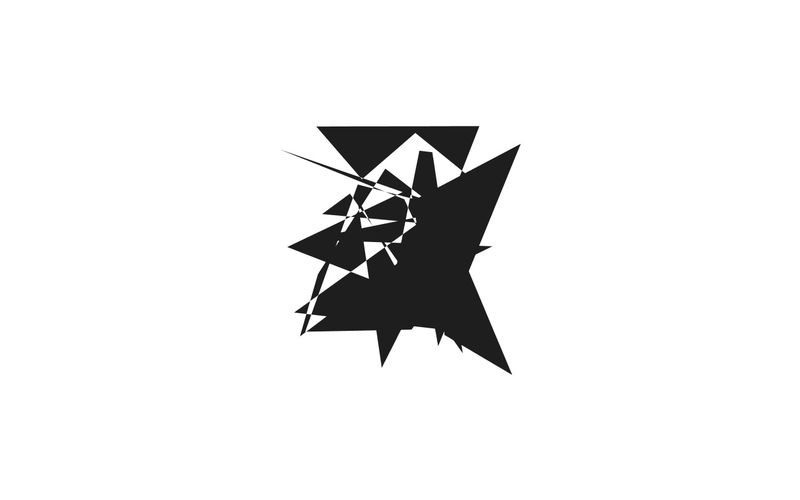See example code

However, that’s not super useful. Instead, we can use the `Math.sin()` and `Math.cos()` functions to create basic and more sophisticated shapes around a center point. They can also help us solve a simple question like this:

How do I find the x and y of a point that is 300 pixels and 33 degrees away from this point?

The same question is illustrated here.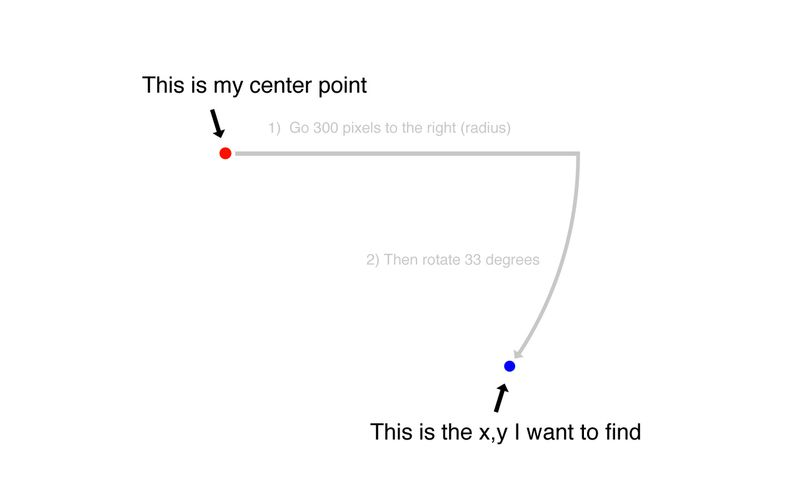To do this we use the `Math.sin()` and `Math.cos()` functions. If you pass in a rotation and multiply it with your radius, they will return the x (cos) and y (sin) for the point we’re trying to find.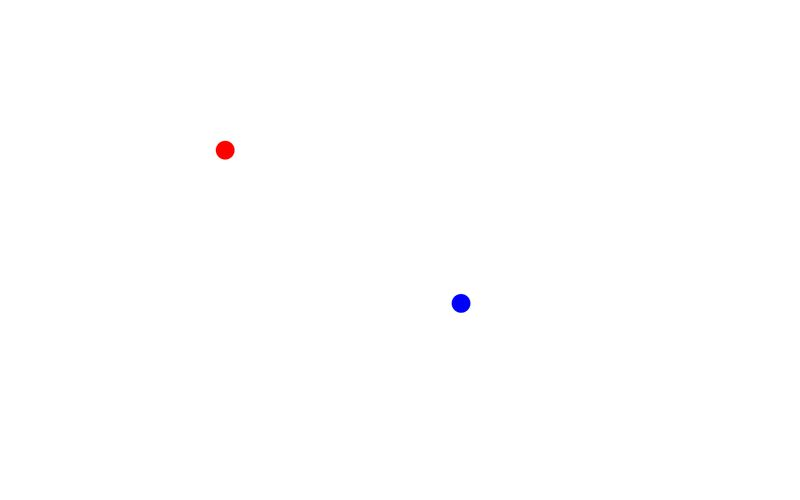See example code

It’s interesting how all the basic shapes (triangle, rectangle, hexagon, circle) can be created with `cos()` and `sin()`, just by changing the number of times the for loop runs.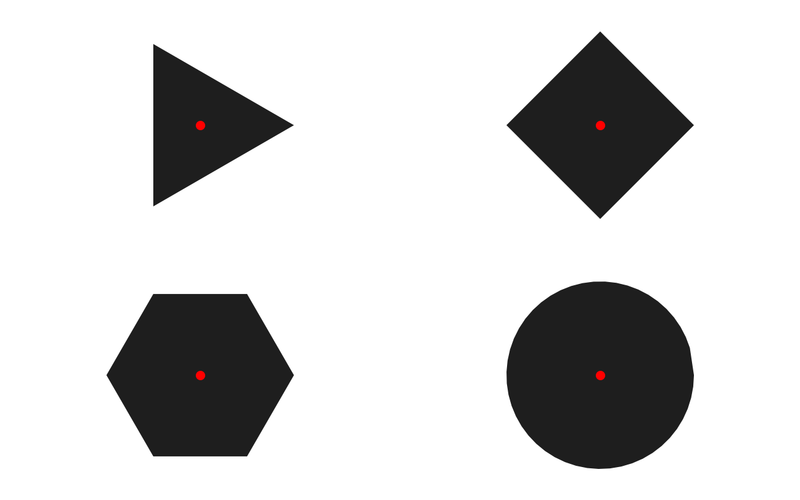See example code

This can be used to put something on the perimeter of a circle. Now we can go back and try to do that in our placement example:

Here’s another sketch that rotates boxes around our center, all oriented towards the center.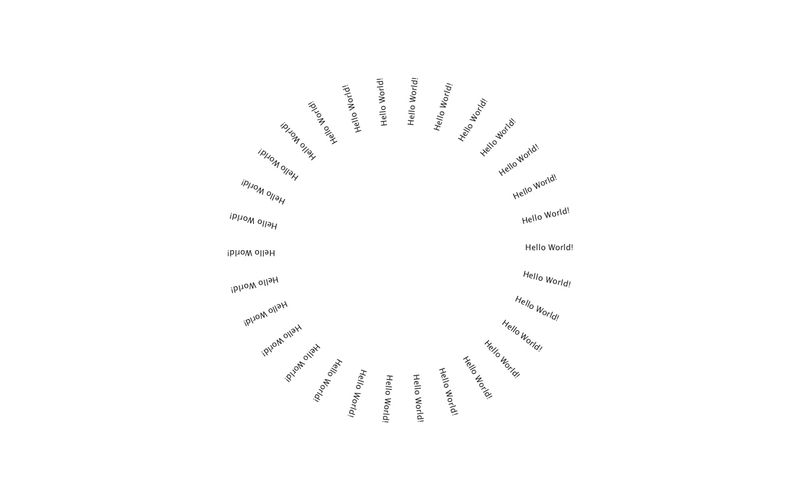See example code

## Paths

Paths are shapes that consist of both straight and curved lines. Please see the `Rune.js` Getting Started document on how to use paths. Also check these simple examples of using quad bezier curves and cubic bezier curves

Bezier curves are essential to computational form, as it’s the way most visual programming languages, as well as software tools like Photoshop and Illustrator (with the Pen tool), approach the drawing of complex polygons. It’s native to the design process of everything from font design to the design of cars.

Although invented by the mathematician Paul de Casteljau, bezier curves got their name from Pierre Bézier who first used these curves in early CAD programs to design cars for Renault.

A cubic bezier curve is a formula that describes how to draw a line from one point to another, using 2 control points. The two control points determine the curve of the line going to that specific point. In Processing, you always start a shape with a simple vertex point, and then draw bezierVertex points after it.

It can be hard to understand how these two control points tell the line to curve like that. This animation shows how the curve of the line is calculated by doing simple vector math on the anchor points and control points.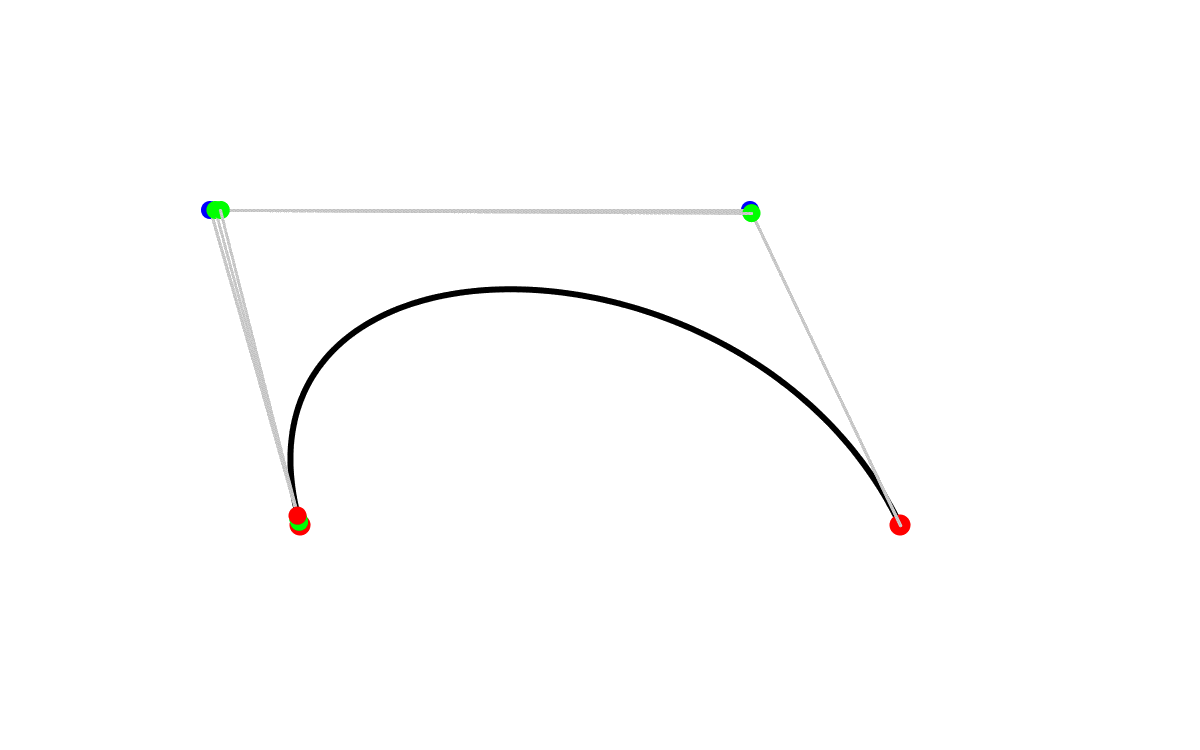Here are some examples with use of more complex forms.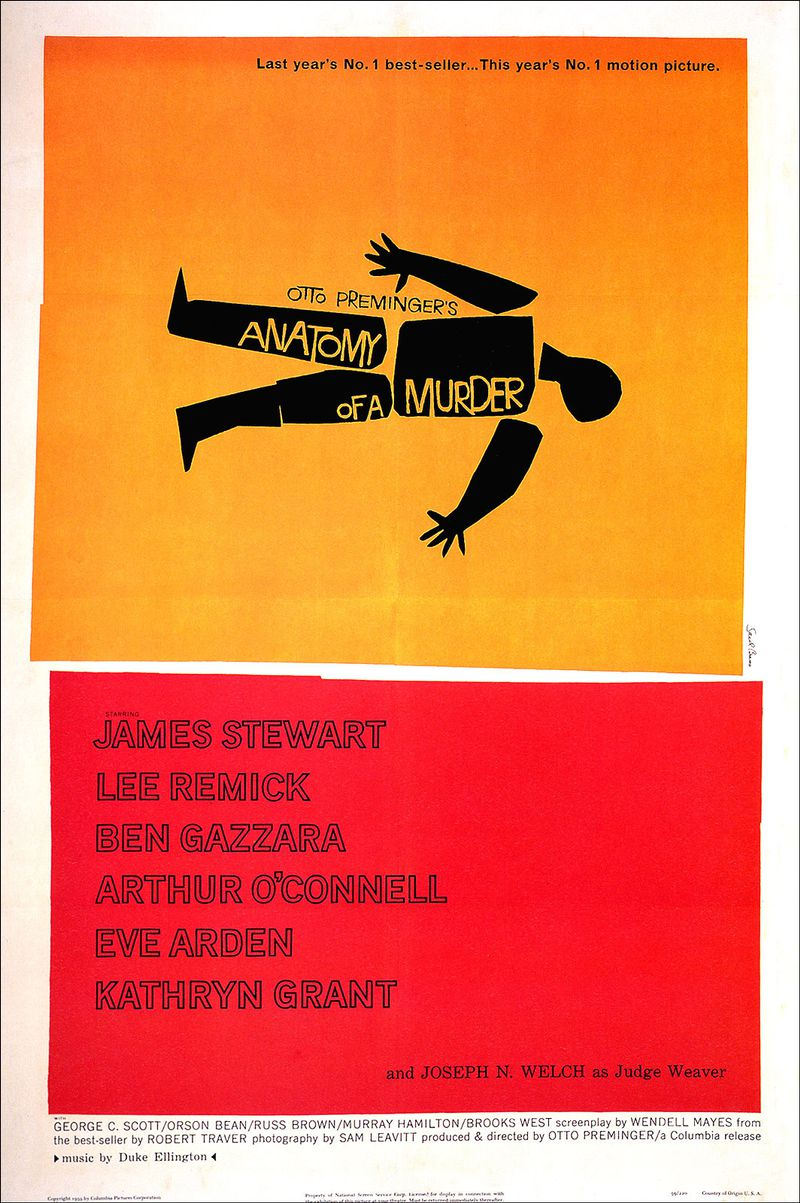Saul Bass’ movie title design is widely know for their simple but very meaningful use of form. Here it’s the movie poster for “Anatomy of a Murder”.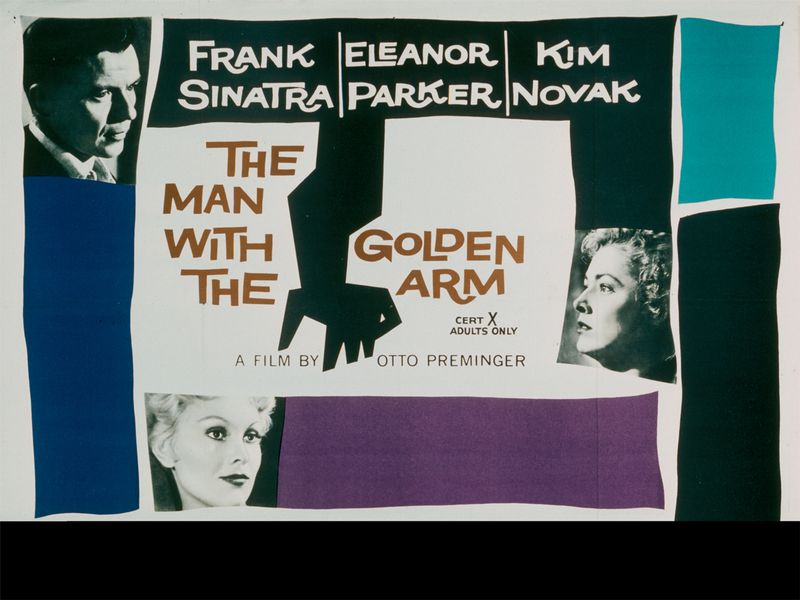Another movie poster by Saul Bass, using the same simple formula.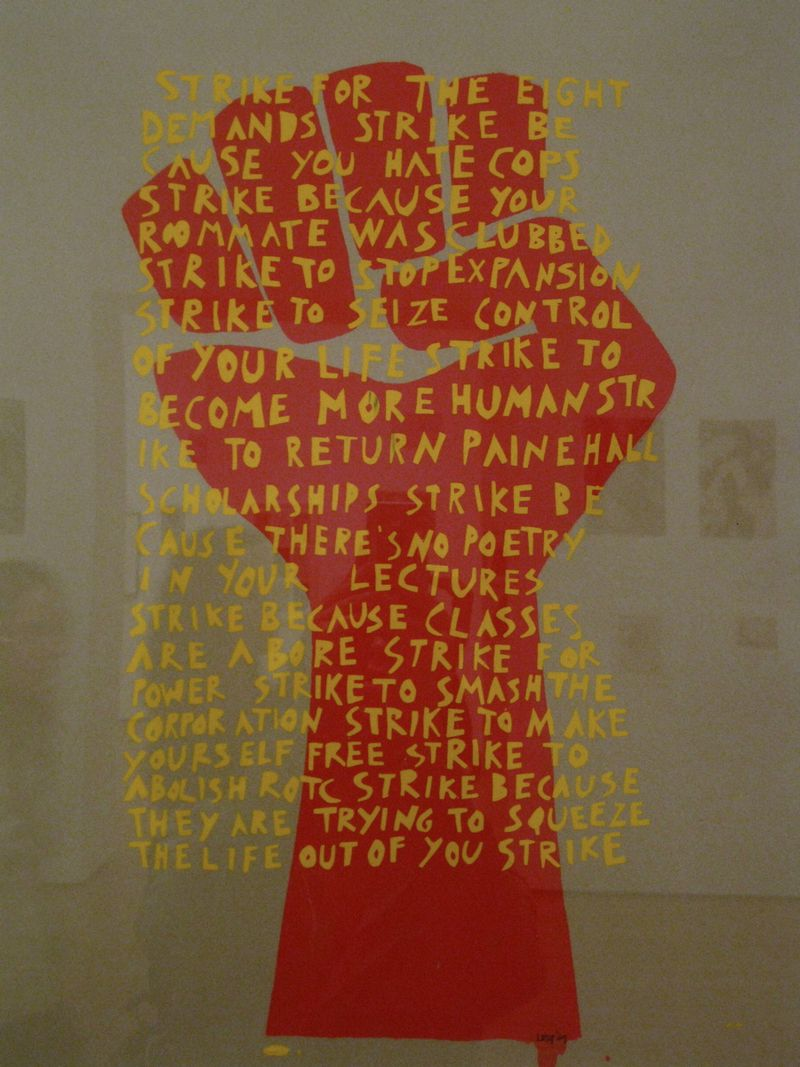The red shape is a very simple collection of polygon, but it still holds tremendous value in its meaning.

## Computational vs. Organic Form

When you start to look at these different shapes, you realize that some of them have inherent algorithmic characteristics. Even Michelangelo will be loose if competing with a computer in drawing a perfect circle. Changing the size of a circle in code is many times faster than doing it by hand.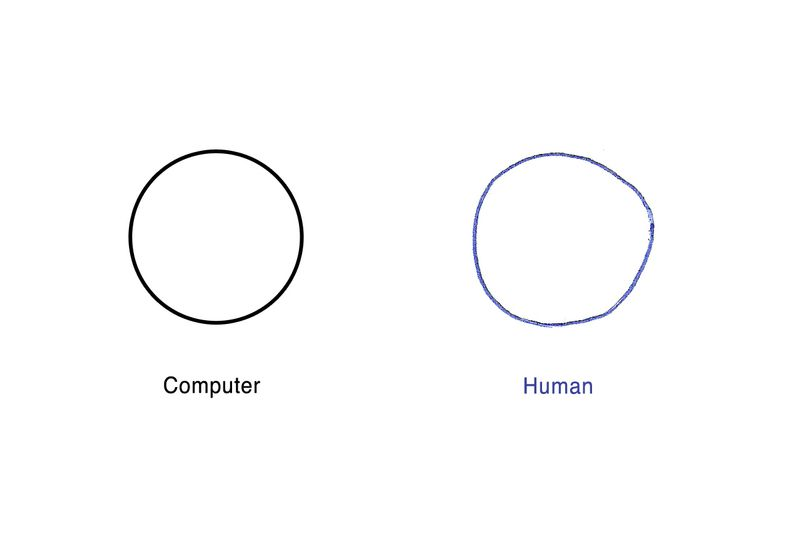Other shapes have inherent organic qualities, and are harder to replicate on a computer. You can draw a random line across a piece of paper in less than one second, but it will take much longer to code it with beginShape().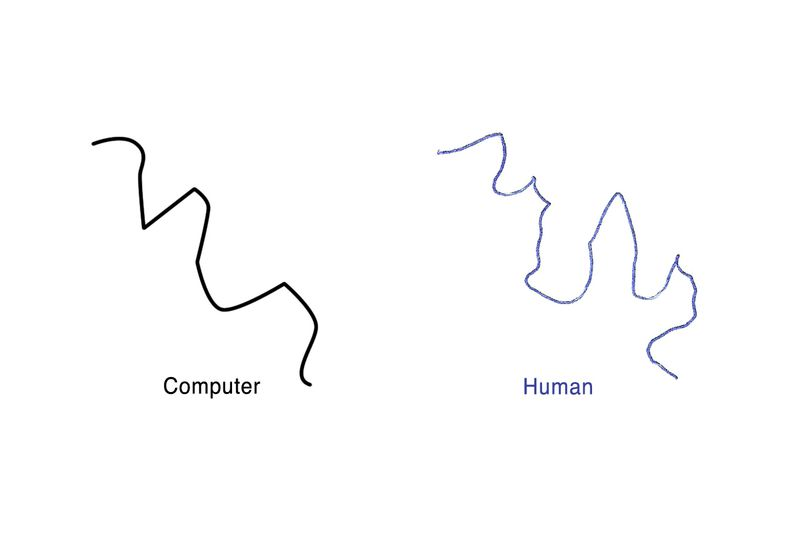This poses a series of questions: When working with computational form, should we prefer some shapes to other? How far should we go to simulate organic, human techniques in the digital world? Is this an argument for a new aesthetic?

## Project Examples and Code

Using these newly learned techniques we can look at a few advanced projects and try to re-create them in Processing.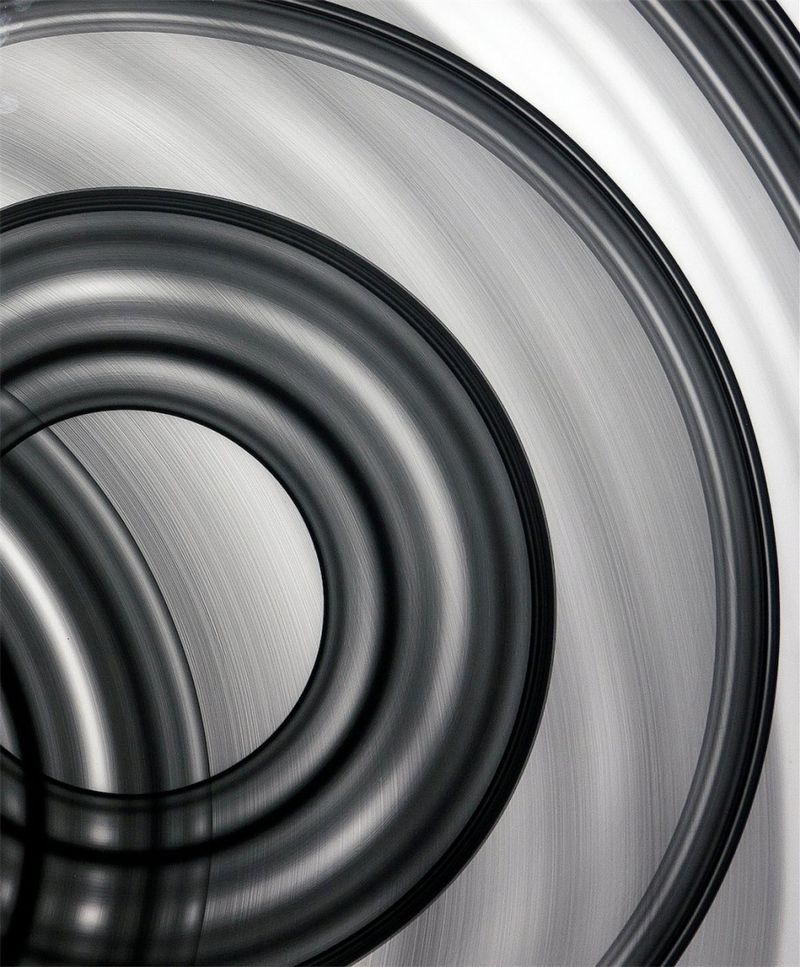Eno Henze is a programming artist from Germany. Here he uses random radius and stroke alpha to create a series of graphic prints.The same visual can be created with Processing using the simple drawing functions, randomization and for loops.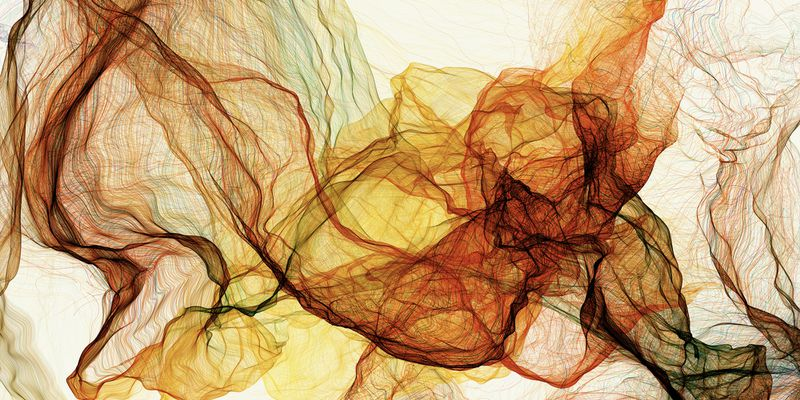Another piece by Eno Henze where he uses simple repetition and random form. This is a great example of the power of using random positioning with vertex points within beginShape().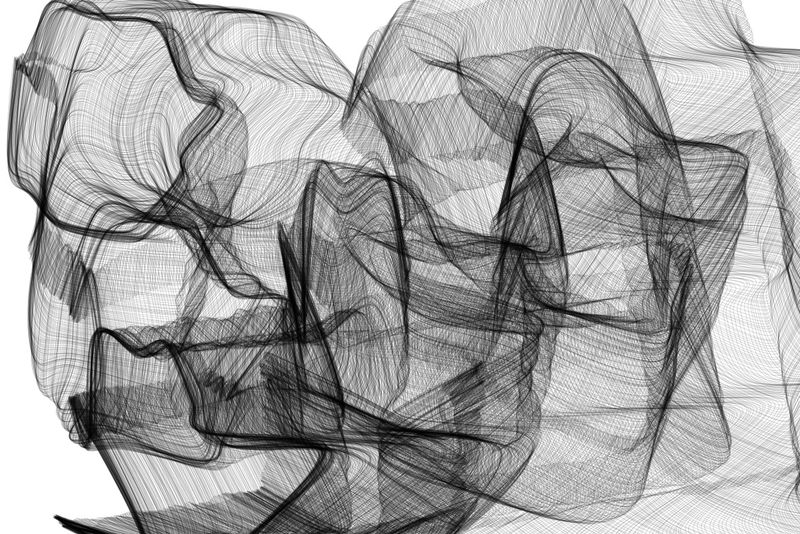See example code

The same visual can be created with Processing and beginShape().